# Supplementary angles

One of the supplementary angles are three times larger than the other. What size is larger of supplementary angles?

a =  135 °

### Step-by-step explanation:

a+b = 180
a = 3b

a+b = 180
a = 3•b

a+b = 180
a-3b = 0

a = 135
b = 45

Our linear equations calculator calculates it.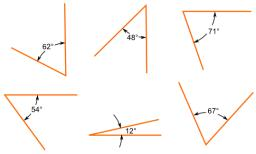Did you find an error or inaccuracy? Feel free to write us. Thank you!Tips to related online calculators
Do you have a linear equation or system of equations and looking for its solution? Or do you have a quadratic equation?

## Related math problems and questions:

• Supplementary angles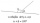One of the supplementary angles is larger by 33° than the second one. Calculate the angles size.
• Angles in ratioThe size of the angles of the triangle are in ratio x: y = 7: 5 and the angle z is 42° lower than the angle y. Find size of the angles x, y, z.
• The second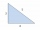The second angle of a triangle is the same size as the first angle. The third angle is 12 degrees larger than the first angle. How large are the angles?
• Obtuse angle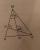The line OH is the height of the triangle DOM, line MN is the bisector of angle DMO. obtuse angle between the lines MN and OH is four times larger than the angle DMN. What size is the angle DMO? (see attached image)
• Angles of the triangleABC is a triangle. The size of the angles alpha, beta are in a ratio 4: 7. The angle gamma is greater than the angle alpha by a quarter of a straight angle. Determine angles of the triangle ABC.
• AngleA straight line p given by the equation y = (-8)/(6) x +78. Calculate the size of angle in degrees between line p and y-axis.
• Supplementary angles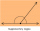(a + 30)° and (2a)° are the measure of two supplementary angles. Find the value of 'a'.
• Alfa, beta, gamaIn the triangle ABC is the size of the internal angle BETA 8 degrees larger than the size of the internal angle ALFA and size of the internal angle GAMA is twice the size of the angle BETA. Determine the size of the interior angles of the triangle ABC.
• Acute angles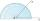Sizes of acute angles in the right-angled triangle are in the ratio 1: 3. What is the size of the larger of them?
• Angles in triangleThe triangle is ratio of the angles β:γ = 6:8. Angle α is 40° greater than β. What are the size of angles of the triangle?
• Acute triangle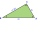In the acute triangle KLM, V is the intersection of its heights and X is the heel of height to the side KL. The axis of the angle XVL is parallel to the side LM and the angle MKL is 70°. What size are the KLM and KML angles?
• Internal anglesOne internal angle of the triangle JAR is 25 degrees. The difference is the size of the two others is 15°. Identify the size of these angles.
• Alfa beta gamaThe triangle's an interior angle beta is 10 degrees greater than the angle alpha and gamma angle is three times larger than the beta. Determine the size of the interior angles.
• In triangleIn triangle ABC, the magnitude of the internal angle gamma is equal to one-third of the angle alpha. The size of the angle beta is 80 degrees larger than the size of the gamma angle. Calculate the magnitudes of the interior angles of the triangle ABC.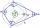What size have angles in quadrilateral (4-gon) if they are in a ratio of 8: 9: 10: 13?The angles α, β, γ in triangle ABC are in the ratio 6:2:6. Calculate size of angles.In a triangle ABC true relationship c is less than b and b is less than a. Internal angles of the triangle are in the ratio 5:4:9. The size of the internal angle beta is: